# Exams Online Testing Platform - Online Exam Platform

## Introduction of the New Version of the Statistics of the Left Menu Bar

Since OnlineExamMaker mainly performed statistical analysis on the exams, and the functions of OnlineExamMaker have been constantly added and updated, the previous statistical analysis has gradually become less applicable.

This month OnlineExamMaker has optimized the [Statistics] module of the system and improved the statistical analysis function. Let’s take a look at the content of the optimized version of the statistical analysis function!

#### New Version of [Statistics]

This new version of statistics is mainly divided into two parts: [Statistics Function] and [Analysis Function]. The statistics part mainly counts the total number of exams and questions in the account; the analysis part is mainly in the form of percentages, such as showing pass rate, test question usage rate, pass rate, etc. The following is a detailed introduction.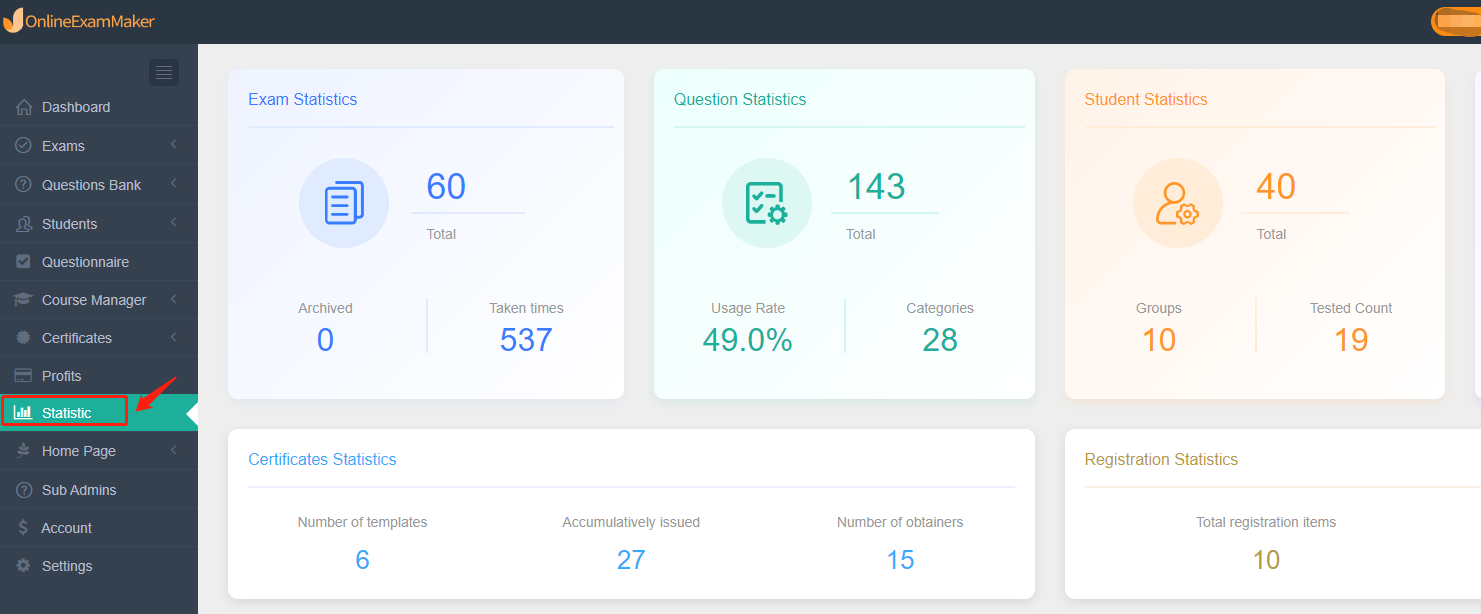##### [Statistics Function]

1.Exam Statistics

Mainly count the total number of exams, the number of times tested, and the number of archived exams.

2.Question Statistics

Mainly count the current total number of questions, question bank usage rate, and total number of categories.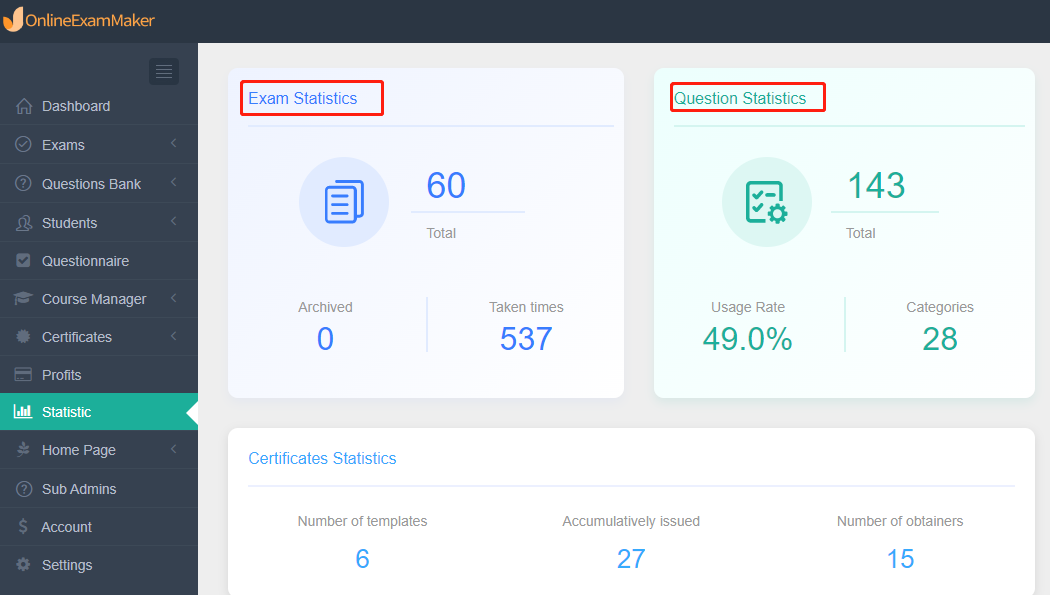3.Student Statistics

The main statistics are the total number of candidates, the total number of groups, and the number of candidates already tested.

4.Prize Quiz Statistics

Mainly count the number of prize quiz created, the total number of categories, and the times of the prize quiz taken.5.Registration Statistics

Including total registration items and number of people registered, users can also click [View details] to know more about the registration information.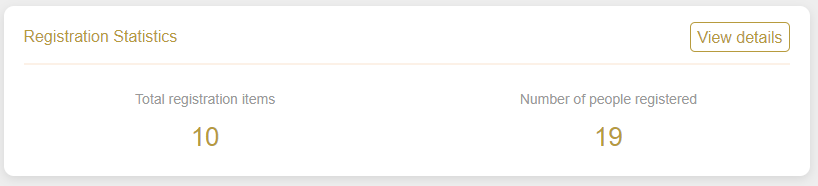##### [Analysis Function]

1.Exam Analysis

It mainly displays exam title, pass times, exam taken times and pass rate. At the same time, click [Details], users can view the statistical analysis of an exact exam.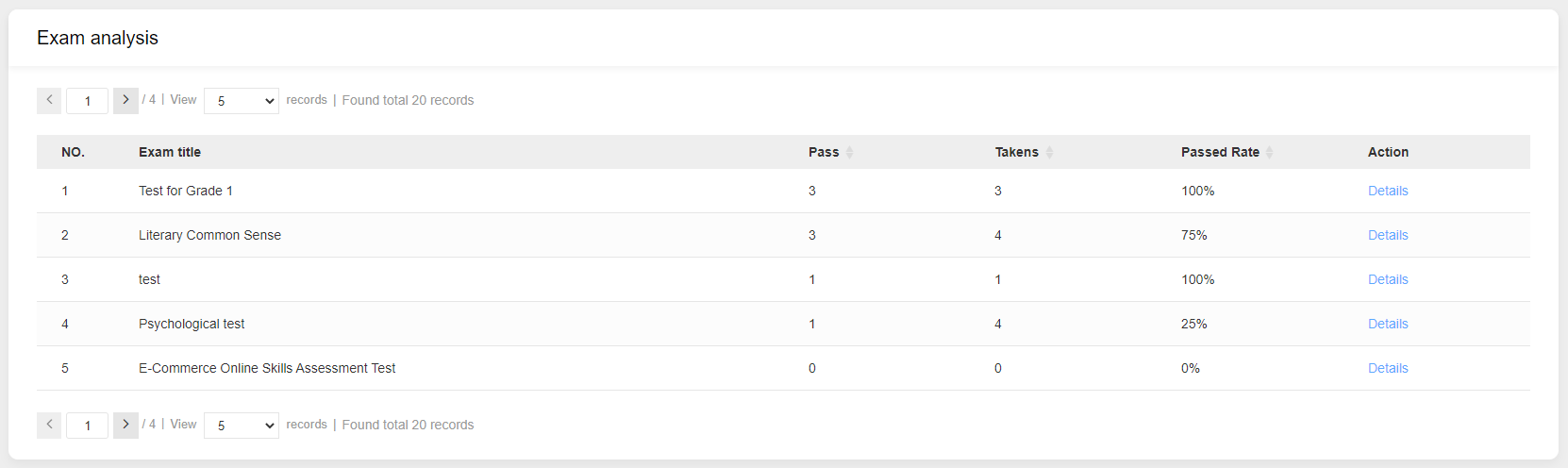2.Question Analysis

Question type statistics: Mainly display the percentage statistics of test question types and the specific quantity statistics of each question type.

Question difficulty statistics: Mainly display the percentage statistics of the difficulty of the test questions and whether the difficulty tag is set.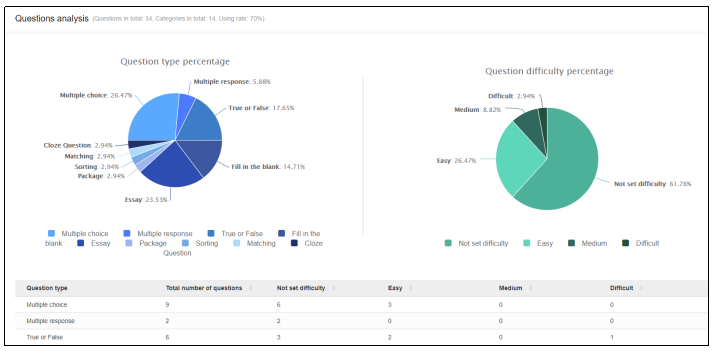3.Student analysis

Count how many candidate groups there are in the system and how many candidates are in each group.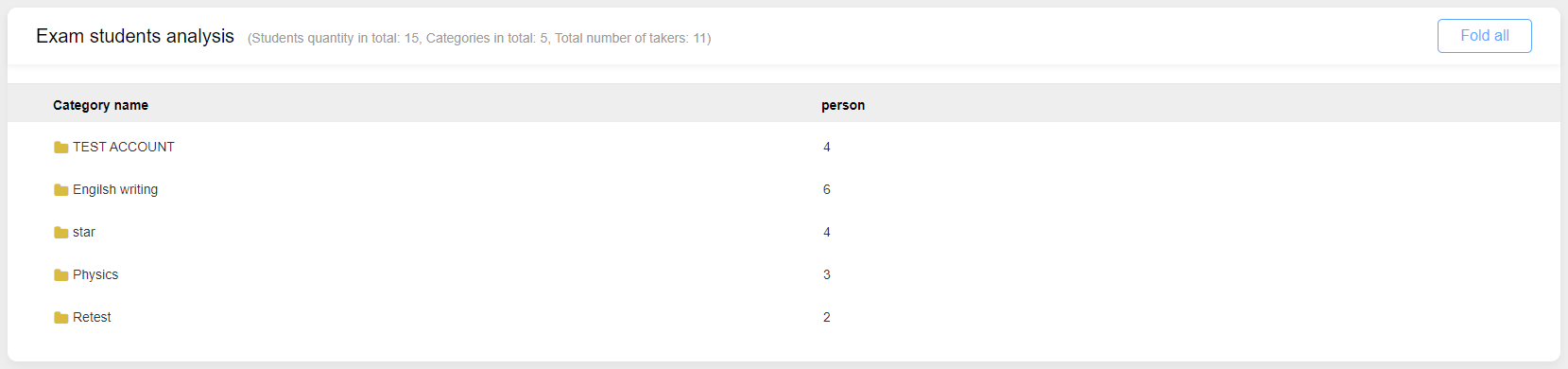4.Analysis of Prize Quiz

List the prize quizzes created in the system, as well as the number of people who have passed the prize quizzes, the times the prize quizzes have been taken, and the pass rate. At the same time, click [Details], users can quickly enter the statistical analysis of a single prize quiz.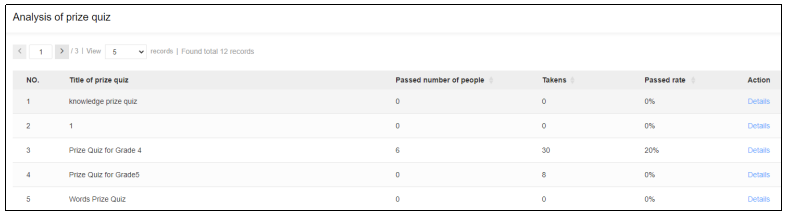This is the introduction of the new version of [Statistics] of OnlineExamMaker. We hope it will meet everyone’s needs for statistical analysis functions!